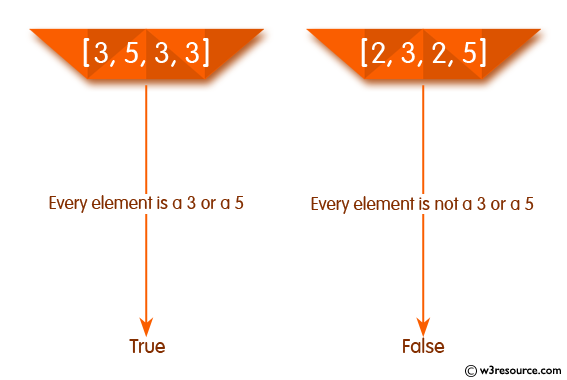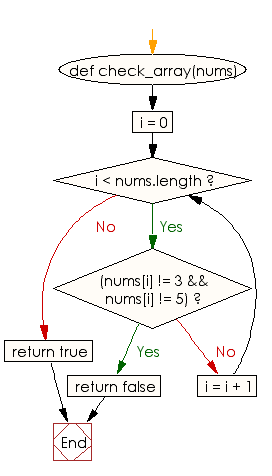﻿ Ruby Array exercises: Check whether every element is a 3 or a 5 in an given array of integers - w3resource# Ruby Array Exercises: Check whether every element is a 3 or a 5 in a given array of integers

## Ruby Array: Exercise-35 with Solution

Write a Ruby program to check whether every element is a 3 or a 5 in a given array of integers.Ruby Code:

``````def check_array(nums)
i = 0
while i < nums.length
if(nums[i] != 3 && nums[i] != 5)
return false
end
i = i + 1
end
return true
end
print check_array([3, 5, 3, 3]),"\n"
print check_array([2, 3, 2, 5]),"\n"
print check_array([3, 5, 5, 5]),"\n"
``````

Output:

```true
false
true
```

Flowchart:Ruby Code Editor: